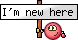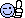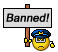Professional Pilot Training (includes ground studies) A forum for those on the steep path to that coveted professional licence. Whether studying for the written exams, training for the flight tests or building experience here's where you can hang out.

# ATPL theory questionsJoin Date: Jan 2006
Location: Europe
Posts: 404
Likes: 0
Received 0 Likes on 0 Posts
Principles of Flight - Flight Mechanics - Forces Acting on Airplane

Hi all,
would need some help with the following two questions:

1. By what percentage does lift increase in a steady level turn at 45° angle of bank, compared to straight and level flight?

2. Twin engine airplane (59.000 Kg) climbing with all engines operating. Lift-Drag ratio is 12. Each engine producing 60.000 N thrust. The gradient of climb is (assume g = 10 m/s˛)?Join Date: Jan 2011
Location: England
Posts: 652
Received 0 Likes on 0 Posts
I think that you will find that there is an error in your first question.

By what percentage does the lift increase in a steady level turn at 45° angle of bank, compared to straight and level flight?

a. 52%.
b. 41%.
c. 19%.
d. 31%.

In order to carry out a constant altitude turn the aircraft must be banked in the direction of the turn. This tilts the lift force towards the lower wing, so that part of it acts horizontally towards the centre of the turn. It is this horizontal component of lift that accelerates the aircraft in the direction of the intended turn.

But tilting the lift force reduces the vertical component of lift, such that it no longer equals the weight of the aircraft. This means that more lift is required in order to prevent the aircraft from sinking. This increase in lift constitutes an increase in load factor.

The load factor at any bank angle in a constant altitude turn is equal to 1 divided by the cosine of the bank angle. So in a 45 degree banked turn the load factor is 1/Cos 45 which is 1/0.7071, or approximately 1.414.

The load factor is equal to the lift divided by the weight. In straight and level flight the lift is equal to the weight of the aircraft and the load factor is 1. So an increase in load factor from 1 to 1.41 means that lift has increased to 141% of its initial value. This means that the lift has increased by 41% (option b).

But had the question been about the change in stalling speed we would have:

By what approximate percentage will the stall speed increase in a horizontal coordinated turn with a bank angle of 45°?

a. 52%.
b. 19%.
c. 31%.
d. 41%.

In order to carry out a constant altitude turn the aircraft must be banked in the direction of the turn. This tilts the lift force towards the lower wing, so that part of it acts horizontally towards the centre of the turn. It is this horizontal component of lift that accelerates the aircraft in the direction of the intended turn.

But tilting the lift force reduces the vertical component of lift, such that it no longer equals the weight of the aircraft. This means that more lift is required in order to prevent the aircraft from sinking. This increase in lift constitutes an increase in load factor.

The load factor at any bank angle in a constant altitude turn is equal to 1 divided by the cosine of the bank angle. So in a 45 degree banked turn the load factor is 1/Cos 45 which is 1/0.7071, or approximately 1.414.

The stall speed in any manoeuvre can be calculated by using the standard equation:

Stall speed at new load factor = 1g stall speed x Square root of (new load factor).

Inserting the data above gives:

Stall speed in 45 degree turn = 1g stall speed x Square root of (1.414) which = 1g stall speed x 1.189.

This means that the stall speed has increased by 18.9% or approximately
19% (option b).

2. Twin engine airplane (59.000 Kg) climbing with all engines operating. Lift-Drag ratio is 12. Each engine producing 60.000 N thrust. The gradient of climb is (assume g = 10 m/s˛)?

If we make the simplifying assumption that lift = weight in a steady climb, then we can calculate climb gradient using the standard equation below.

% Gradient = 100 x ( (thrust/weight) – (1/Lift: Drag ratio)).

This can also be expressed as:

% Gradient = 100 x ( (thrust/weight) – (Drag/Lift)).

It is essential that both all forces are expressed in the same units.
In this question weight is in kg and thrust is in Newton, so one must be of these must be converted to match the other.
To convert Newton to kg divide by g.
So thrust per engine = 60000 N /10 m/s/s = 6000 kg thrust.

The question asks for the all engines climb gradient so the total thrust is 2 x 6000 = 12000 kg.

Inserting the data into the equation gives:
% Gradient = 100 x ( (thrust/weight) – (Drag/Lift) ).
% Gradient = 100 x ( (12000 kg /59000 kg) – (1/12)).

Last edited by keith williams; 31st Jan 2013 at 20:00.Join Date: Nov 2009
Location: PK
Posts: 195
Likes: 0
Received 0 Likes on 0 Posts
Hello Keith

Have you seen this one:

The range to a required waypoint presented by RNAV system is:

plan range or slant range depending on RNAV settings
plan range
slant range
neither plan range nor slant range

Isnt it always plan range that is presented?

RegardsJoin Date: Jan 2011
Location: England
Posts: 652
Received 0 Likes on 0 Posts
I have not seen the question before, so I cannot say with any certainty what the examiner's answer is.

If the RNAV system is using DME as an input then this will introduce slant range errors.

I believe that modern RNAV systems compensate for slant range errors, but earlier systems probably do not do so.

Last edited by keith williams; 1st Feb 2013 at 10:26.Join Date: Nov 2009
Location: PK
Posts: 195
Likes: 0
Received 0 Likes on 0 Posts
Thanks Keith, the examiner answer is highlighted in bold.

anyway thanks a lot.

Last edited by Haroon; 1st Feb 2013 at 10:57.Join Date: Jan 2006
Location: Europe
Posts: 404
Likes: 0
Received 0 Likes on 0 Posts
Keith, thanks a lot for taking the time to answer my questions in such detailed manner!!!! Very much appreciated!!!! Your explanation helped me a lot, it now appears to be so simple and crystal clear. People like you and your competent posts really contribute to enhance this forum!Join Date: Jan 2011
Location: England
Posts: 652
Received 0 Likes on 0 Posts
Happy to be of help Transsonic 2000.

Most of my explanations are taken from my range of books and CDs, so in most cases providing them here involves little extra work. It's mainly a copy-paste job.

Last edited by keith williams; 2nd Feb 2013 at 09:47.Join Date: Jan 2006
Location: Europe
Posts: 404
Likes: 0
Received 0 Likes on 0 Posts
Hi there,
having trouble figuring out the following QDB questions, would appreciate if someone could shed some light on it:

1. Whilst maintaining straight & level flight with a lift coefficient of CL=1, what will be the new value of CL after the speed is increased by 41%:

a) 0.25
c) 0.60
d) 0.30

2. Airplane flying at 100 kt in straight & level flight is subject to a disturbance that suddenly increases the speed by 20 kt. Assuming AoA remains constant, load factor will initially be:

a) 1.41
c) 1.30
d) 1.04

3. In straight & level flight at a speed of 1.3 VS, the lift coefficient, expressed as a percentage of of its maximum CLMAX, would be:

a) 169%
b) 130%
d) 77%

Help appreciated, thanks a lot!Join Date: Jan 2011
Location: England
Posts: 652
Received 0 Likes on 0 Posts
1. Whilst maintaining straight & level flight with a lift coefficient of CL=1, what will be the new value of CL after the speed is increased by 41%:

a) 0.25
c) 0.60
d) 0.30

Lift = Cl 1/2Rho V squared S

Where V is the TAS.

If speed increase by 41% it becomes 1.41 of its initial value.

So V squared becomes approximate 2 times its initial value.

The question states that SL flight is to be maintained, so the lift must not change.

So to maintain level flight the Cl must become ˝ of its initial value.

2. Airplane flying at 100 kt in straight & level flight is subject to a disturbance that suddenly increases the speed by 20 kt. Assuming AoA remains constant, load factor will initially be:

a) 1.41
c) 1.30
d) 1.04

Lift = Cl 1/2Rho V squared S

Where V is the TAS.

Cl is proportional to AoA, so if AoA remains unchanged then Cl remains unchanged.

The 20 kt increase from 100 kts increases speed to 120 kts. This is 1.2 times its initial value.
So V squared becomes 1.2 squared = 1.44 times its initial value.

So the new lift = 1.44 times its initial value.

If the aircraft was initially in SL flight the lift must have been equal to the weight and the load factor was Lift / weight = 1

So if we use 1 to represent the initial lift we can use 1.44 to represent the lift in the gust.

So in the gust the load factor = 1.44 / 1 = 1.44

3. In straight & level flight at a speed of 1.3 VS, the lift coefficient, expressed as a percentage of of its maximum CLMAX, would be:

a) 169%
b) 130%
d) 77%

In SL flight at any speed above Vs
Lift = Cl 1/2Rho V squared S

At the stall in SL flight
Lift = ClMax 1/2Rho Vs squared S

Assuming weight is unchanged in the two equations above we can say that the first equation is equal to the second.

If we take out the common factors of Lift and 1/2Rho S we leave the following:

Cl V squared = ClMax Vs squared

Rearranging this gives us

Cl = ClMax Vs squared / V squared)

If we use 1 to represent Vs we can use 1.3 to represent 1.3Vs. Putting these values into the equation gives us
Cl = ClMax x (1 squared / 1.3 squared)

Cl = ClMax x (1 / 1.69)

Cl = 0.59 17 ClMax.

This is approximately 59% of Cl Max

For this type of question just remember the following

Cl at any speed = ClMax x ( Vs squared /Speed chosen squared )

Last edited by keith williams; 4th Feb 2013 at 09:03.Join Date: Jan 2006
Location: Europe
Posts: 404
Likes: 0
Received 0 Likes on 0 Posts
Keith, once again I must say thanks a lot! Your explanations are really outstanding, it makes things appear easy and simple to understand. I see myself ending up buying one of your booksLast edited by Transsonic2000; 4th Feb 2013 at 11:30.Join Date: Jun 2007
Location: SAYE
Posts: 279
Likes: 0
Received 0 Likes on 0 Posts
but only for locals ???

Please note: Access will be limited to users operating in/from South African Air Space, for aviation purposes only. Although not all the fields are mandatory we require RSA contact details and pilot licence number - add license number in the motivation! If you are a handler or operator for an airline please state this in the motivationJoin Date: Feb 2013
Location: Amsterdam
Posts: 6
Likes: 0
Received 0 Likes on 0 Posts
Jammed

Hi,

Could anybody tell me what a ''jammed'' control column or elevator is?

Thanks.Join Date: Dec 2006
Location: Hamburg
Age: 45
Posts: 430
Likes: 0
Received 0 Likes on 0 Posts
'Jammed' means stuck, unable to move, e.g. because someone in the cockpit has dropped something that blocks the control column or because of ice accretion on the elevator.Join Date: Nov 2009
Location: PK
Posts: 195
Likes: 0
Received 0 Likes on 0 Posts
flux valve

A flux valve detects the horizontal of the earth’s magnetic field

1) the flux valve is made of a pair of soft iron bars
2) the information can be used by a “flux gate” compass or a directional gyro
3) the flux gate valve signal comes from the error detector
4) the accuracy on the value of the magnetic field indication is less than 0.5%

Which of the following combinations contains all of the correct statements?

a) 2, 4
b) 1, 2, 4
c) 1, 4
d) 1, 2

a) marked correct. Why not (b)?

can some also pls explain the meaning of "the accuracy on the value of the magnetic field indication is less than 0.5%"

this seems pretty inaccurate

thanksJoin Date: Apr 2009
Location: down south
Age: 76
Posts: 13,226
Likes: 0
Received 0 Likes on 0 Posts
'b' is incorrect because a flux valve assembly comprises three pairs of cores at 120 degrees to each other.Join Date: Nov 2009
Location: PK
Posts: 195
Likes: 0
Received 0 Likes on 0 Posts
thanks lightning mate. I had a feeling it might be that but never thought they'll dig in that deep.

Do you have any idea abut this one:

the accuracy on the value of the magnetic field indication is less than 0.5%

thanks a lot

Last edited by Haroon; 12th Mar 2013 at 03:30.Join Date: Apr 2009
Location: down south
Age: 76
Posts: 13,226
Likes: 0
Received 0 Likes on 0 Posts
I have no idea what that means.

The highest accuracy in any system can only be 100%, so less than 0.5% is very very poor.

I don't know where you found the question, but I taught the JAA syllabus for twenty years, including some time setting and verifying questions in the CQB at Gatwick.

I know of many flawed/invented questions in commercial so-called "databases".

Beware.Join Date: Nov 2009
Location: PK
Posts: 195
Likes: 0
Received 0 Likes on 0 Posts
Thanks LM

I know of many flawed/invented questions in commercial so-called "databases".
probably this one is a victim as well unless they mean "inaccuracy"

regardsJoin Date: Jun 2012
Location: Ballarat
Posts: 10
Likes: 0
Received 0 Likes on 0 Posts
Stalls exam question right or wrong?

The question
During a climb at maximum power, the IAS is progressively reduced from the maximum rate of climb speed to the stalling speed.
The effect on the Angle of climb is that it will..

A)Angle of Climb will Increase Continuously
B)Angle of Climb will Decrease Continuously
C)Angle of Climb will Increase then Decrease
D)Angle of Climb will Decrease then Increase

I thought considering the stalling IAS with power on would be lower then the actual published Stall speed of the Aircraft the answer would have to be that the Angle would increase continously.. but am I wrong in assuming the question would factor in that the stall speed would be at a lower IAS then published due to power?

Last edited by outbacktourer; 13th Mar 2013 at 04:30.Join Date: Jan 2011
Location: England
Posts: 652
Received 0 Likes on 0 Posts
You are missing the point of the question.

Vx is greater than Vs.
Vx is the speed at which angle of climb is best.
Vy is the speed at which rate of climb is best.
Vy is greater than Vx.

So our sped range is

Low speed...Vs.............Vx.............Vy...........High speed

In this question we are decelerating from Vy (at which ROC is best), through Vx (at which angle of climb is best) to Vs (at which we stall).

This will cause the angle of climb to increase (until we reach Vx) then to decrease (until we stall).Show Printable VersionEmail this Page